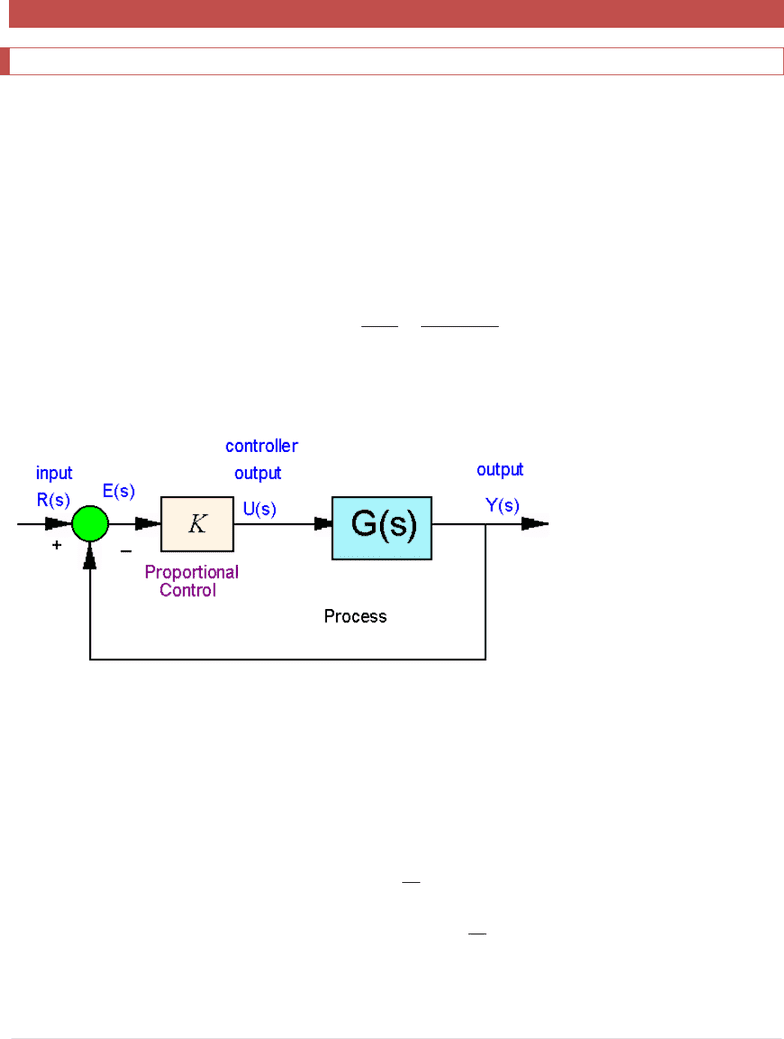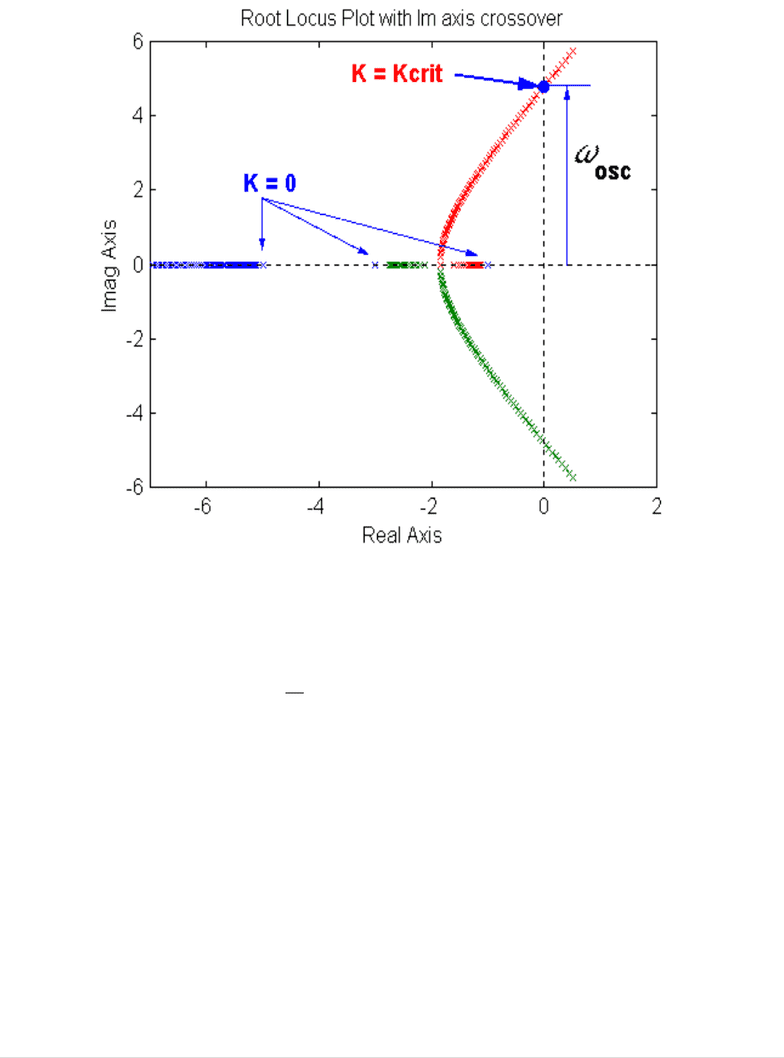# ELE 639 Lecture Notes - Lecture 10: Root Locus, Algebraic Equation, Imaginary Number

14 views25 pages
School
Course
ProfessorELE639 Course Notes Winter 2018
182 | P a g e
10 Root Locus Analysis
10.1 Introduction
The Root Locus method is a graphical technique used to plot the location of the poles of a closed loop system as
one of the system parameters is varied. This technique is used to provide a measure of the relative stability of a
system, as well as to determine appropriate parameter values, which will yield suitable root locations. In the
general case the open loop transfer function is G(s)H(s). However, typically an equivalent open loop system is
analyzed, with H(s) = 1. Root Locus equations below are derived for such a case, without a loss of generality (see
the notes on the equivalent unit feedback loop).
Consider a simple unity feedback system, such as in Figure 10-1, with the variable proportional controller gain K.
The closed loop system transfer function and its characteristic equation are as follows:
0)(1
)(1
)(
)(
)(
)(
sKG
sKG
sKG
sR
sY
sGcl
Figure 10-1 Closed Loop Equivalent Unit Feedback System under Proportional Control
The location of closed loop poles can be traced by plotting them on the same plot for different values of
proportional gain K, as shown in Figure 10-2. The resulting plot is called the Root Locus plot. The Root Locus
technique can be used to an s-domain based analysis and design of control systems.
The poles of the closed loop system are determined by solving the characteristic equation:
K
sGsG
K
sG
sKG
1
)(|)(|
1
)(
0)(1
Equation 10-1
Unlock document

This preview shows pages 1-3 of the document.
Unlock all 25 pages and 3 million more documents.ELE639 Course Notes Winter 2018
183 | P a g e
Figure 10-2 Example of a Root Locus Plot
Therefore, for the points in S-domain to belong to the Root Locus, it is necessary that two equations be satisfied:
)(360180)(
)(
1
|)(|
criterionanglensG
criterionmagnitude
K
sG
Equation 10-2
So, for any gain K, we can solve the above equations to determine the closed loop system pole locations. Notice, if
we allow the controller gain to vary, i.e.,
K0
, and solve the above two equations, we can plot the
resulting locations (loci) of the closed loop system poles for all (positive) choices of controller gain K, as shown in
Figure 10-2.
Unlock document

This preview shows pages 1-3 of the document.
Unlock all 25 pages and 3 million more documents.ELE639 Course Notes Winter 2018
184 | P a g e
10.2 Evans' Root Locus Construction Rules - Introduction
To use analytic techniques to solve Equation 10-2 for
K0
, is time consuming, since we would have to
tediously solve and plot the resulting magnitudes and phase angles of G(s) that satisfy the magnitude and phase
criteria. Also, note that no formulae exist for roots of polynomials of order higher than third, and the roots for
polynomials of higher orders have to be found using iterative numerical methods, such as Newton-Raphson.
However, as it turns out, such analytical solutions are not necessary because Root Loci follow a set of simple
construction rules that were formulated in 1948 by Walter R. Evans, who was working in the field of guidance and
control of aircraft. These rules stem from Equation 10-2 that must be satisfied for every point on the Root Locus,
and constitute an orderly process for sketching an approximation of the root loci for
K0
.
Evans' Root Locus construction method utilizes the graphical evaluation of a function in the s-plane:
for any point in the s-plane, the open loop function
 
*
sG
can be evaluated;
the angle criterion for
 
*
sG
can be checked;
if the angle criterion is met, the point
*
s
belongs to a system Root Locus.
Construction rules developed by Evans dealt with:
Rule 1: Beginning and end of Root Locus plot, symmetry;
Rule 2: Points on the Real axis;
Rule 3: Asymptotic angles and centroid;
Rule 4: Break-away (break-in) points;
Rule 5: Crossovers with Imaginary axis;
Rule 6: Angles of departure (arrival) from/to complex poles (zeros).
Evans also established how to determine the Proportional Gain used in the closed loop system operation that
corresponds to any particular point
on the Root Locus by:
Preparing an accurate Root Locus plot
Using the magnitude criterion to evaluate the gain at the point
*
s
|)(|
1
0)(1 *
***
sG
KsGK
Unlock document

This preview shows pages 1-3 of the document.
Unlock all 25 pages and 3 million more documents.

## Document Summary

The root locus method is a graphical technique used to plot the location of the poles of a closed loop system as one of the system parameters is varied. This technique is used to provide a measure of the relative stability of a system, as well as to determine appropriate parameter values, which will yield suitable root locations. In the general case the open loop transfer function is g(s)h(s). However, typically an equivalent open loop system is analyzed, with h(s) = 1. Root locus equations below are derived for such a case, without a loss of generality (see the notes on the equivalent unit feedback loop). Consider a simple unity feedback system, such as in figure 10-1, with the variable proportional controller gain k. The closed loop system transfer function and its characteristic equation are as follows: sgcl sy sr skg skg. Figure 10-1 closed loop equivalent unit feedback system under proportional control.

## Get access

\$8 USD/m\$10 USD/m
Billed \$96 USD annuallyHomework Help
Study Guides
Textbook Solutions
Class Notes
Textbook Notes
Booster Class